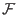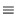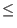Next: Steps to Shor's Algorithm Up: Quantum Algorithms Previous: Motivation for Shor's Algorithm   Contents

## Overview of Shor's Algorithm

Shor's algorithm hinges on a result from number theory. This result is:

The function(a) = xa mod n is a periodic function, where x is an integer coprime to n. In the context of Shor's algorithm n will be the number we wish to factor. When two numbers are coprime it means that their greatest common divisor is 1.

Calculating this function for an exponential number of a's would take exponential time on a classical computer. Shor's algorithm utilizes quantum parallelism to perform the exponential number of operations in one step.

The reason why this function is of utility in factoring large numbers is this:

Since(a) is a periodic function, it has some period r. We know that x0 mod n = 1, so xr mod n = 1, and x2r mod n = 1 and so on since the function is periodic.

Given this information and through the following algebraic manipulation:

xr1 mod n

(xr/2)2 = xr1 mod n

(xr/2)2 -10 mod n

and if r is an even number

(xr/2 -1)(xr/2 +1)0 mod n

We can see that the product (xr/2 -1)(xr/2 + 1) is an integer multiple of n, the number to be factored. So long as xr/2 is not equal to1, then at least one of (xr/2 - 1) or (xr/2 + 1) must have a nontrivial factor in common with n. So by computing gcd(xr/2 - 1, n), and gcd(xr/2 + 1, n), we will obtain a factor of n, where gcd is the greatest common denominator function.

Here is a brief overview of Shor's algorithm, which is explained in detail in the next section. Shor's algorithm tries to find r, the period of xa mod n, where n is the number to be factored and x is an integer coprime to n. To do this Shor's algorithm creates a quantum memory register with two parts. In the first part the algorithm places a superposition of the integers which are to be a's in the xa mod n function. We will choose our a's to be the integers 0 through q - 1, where q is the power of two such that n2q < 2n2. Then the algorithm calculates xa mod n, where a is the superposition of the states, and places the result in the second part of the quantum memory register.

Next the algorithm measures the state of the second register, the one that contains the superposition of all possible outcomes for xa mod n. Measuring this register has the effect of collapsing the state into some observed value, say k. It also has the side effect of projecting the first part of the quantum register into a state consistent with the value measured in the second part. Since we have partitioned our quantum register into two parts measurement of the second part collapses that part into exactly one value, while the other partition collapses into a state consistent with the observed value in the other portion of the register. It is still possible for the non measured part of the register to exist in a superposition of base states, as long as each of those base states are consistent with the measured value in the other part of the register. What this means in this instance is that after this measurement the second part of the register contains the value k, and the first part of the register contains a superposition of the base states which when plugged into xa mod n produce k. Since we know xa mod n is a periodic function, we know that the first part of the register will contain the values c, c + r, c + 2r... and so on, where c is the lowest integer such that xc mod n = k.

The next step is to perform a discrete Fourier transform on the contents of first part of the register (the one containing all integers such that xa mod n = k) and to put the result back into register one. The application of the discrete Fourier transformation has the effect of peaking the probability amplitudes of the first part of the register at integer multiples of the quantity q/r.

Now measuring the first part of the quantum register will yield an integer multiple of the inverse period. Once this number is retrieved from the quantum memory register, a classical computer can do some analysis of this number, make a guess as to the actual value of r, and from that compute the possible factors of n. This post processing will be covered in more detail later. (Shor)Next: Steps to Shor's Algorithm Up: Quantum Algorithms Previous: Motivation for Shor's Algorithm   Contents
Matthew Hayward - Quantum Computing and Shor's Algorithm GitHub Repository